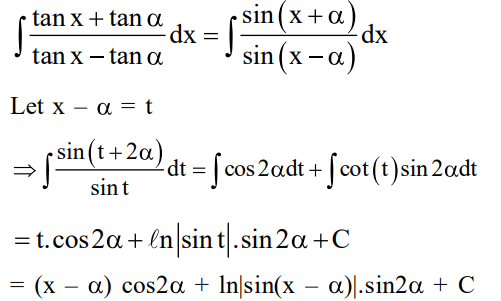# Let α ∈ (0 , π/2) be fixed.`
Question:

Let $\alpha \in(0, \pi / 2)$ be fixed. If the integral

$\int \frac{\tan x+\tan \alpha}{\tan x-\tan \alpha} d x=$

$A(x) \cos 2 \alpha+B(x) \sin 2 \alpha+C$, where $C$ is a constant of integration, then the functions $A(x)$ and $B(x)$ are respectively :

1. $x-\alpha$ and $\log _{e}|\cos (x-\alpha)|$

2. $x+\alpha$ and $\log _{e}|\sin (x-\alpha)|$

3. $\mathrm{x}-\alpha$ and $\log _{\mathrm{e}}|\sin (\mathrm{x}-\alpha)|$

4. $x+\alpha$ and $\log _{e}|\sin (x+\alpha)|$

Correct Option: , 3

Solution: